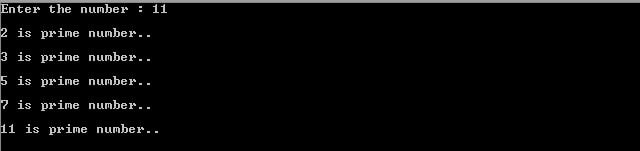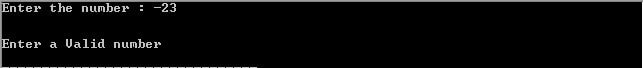# C Programs to find all prime number upto n

Previously we have read how to find prime number using C code, here we will learn how to find the all prime number up to n.

A prime number is a positive natural number, whose value greater than 1 and it has only two factors 1 and the number itself. Either you can say that prime numbers only divided by itself and 1.

There are many ways to find all prime numbers up to n. In this blog post, we will discuss the Trial division method and the Sieve of Eratosthenes algorithm.

Let us see an example to understand the sentence “prime numbers up to n”.

Suppose a given number is n, the task is to print all prime numbers up to n. So if the user enters 10 then the output will be 2,3,5,7.

### Trial Division Method

#### Example 1.

It is the simplest way to find the all prime numbers of an integer n. In this method, we are using two loops outer and nested. The outer loop is used to produce the numbers up to “n” and the nested loop is used to check the numbers for the prime number. If any of the numbers are prime then nested loop print this number.

#include<stdio.h>
int main(int argc, char *argv[])
{
int iRetValue = 0;
int iNumber = 0;
int iLoop =0;
int iLoopin =0;
int iLimit =0;
int iPrimeFlag = 0;
printf(“Enter the number : “);
scanf(“%d”,&iNumber);
if( iNumber <= 1)
{
printf(“\n\nEnter a Valid number\n”);
return 0;
}
else
{
//outer loop to create the number
for(iLoop=2 ; iLoop <= iNumber; iLoop++)
{
iPrimeFlag = 0; // Assign value to flag
// Calculate the limit for nested loop
iLimit = iLoop/2;
for(iLoopin = 2; iLoopin <= iLimit; iLoopin++)
{
// Check prime number
if((iLoop % iLoopin) == 0)
{
iPrimeFlag = 1;
break;
}
}
if(iPrimeFlag == 0) //Print if number is prime
{
printf(“\n %d is a prime number..\n”, iLoop);
}
}
}
return 0;
}

Output of the program##### Example 2.

In this method, we are using a loop and function to find all prime numbers of an integer. The loop is used to create numbers up to n and function is used to check the number prime or not. If the number is a prime number then the function return “1” either its returns “0”.

#include <stdio.h>
#define PRIME_NUMBER 1
{
int iLoop = 0;
int iPrimeFlag = 1;
//Divide the number by 2
int iLimit = iNumber/2;
for(iLoop = 2; iLoop <= iLimit; iLoop++)
{
if((iNumber % iLoop) == 0) // Check prime number
{
iPrimeFlag = 0;
break;
}
}
return iPrimeFlag;
}
int main(int argc, char *argv[])
{
int iRetValue = 0;
int iNumber = 0;
int iLoop =0;
printf(“Enter the number : “);
scanf(“%d”,&iNumber);
if( iNumber <= 1)
{
printf(“\n\nEnter a Valid number\n”);
return 0;
}
else
{
for(iLoop=2 ; iLoop <= iNumber; iLoop++)
{
//Check Prime Number
if (iRetValue == PRIME_NUMBER)
{
printf(“\n%d is prime number..\n”, iLoop);
}
}
}
return 0;
}

Output of the above program